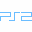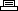Site SearchOver The HedgePrinter Friendly Version

```Cheat mode:
Press Start to pause game play, then enter one of the following codes at
the pause screen.

Level select:
Hold L1 + R1 and press Triangle, Circle, Triangle, Circle(2), Square.

All mini-games:
Hold L1 + R1 and press Triangle, Circle, Triangle(2), Square(2).

All moves:
Hold L1 + R1 and press Triangle, Circle, Triangle, Square(2), Circle.

Always power golf:
Hold L1 + R1 and press Triangle, Circle, Triangle, Circle, Square,
Circle.

More HP from food:
Hold L1 + R1 and press Triangle, Circle, Triangle, Circle, Square,
Triangle.

Extra damage:
Hold L1 + R1 and press Triangle, Circle, Triangle, Circle, Triangle,
Square.

Bonus comic 1:
Hold L1 + R1 and press Triangle, Circle, Square(2), Circle, Triangle.

Bonus comic 2:
Hold L1 + R1 and press Triangle(2), Square, Circle, Square, Circle.
```